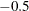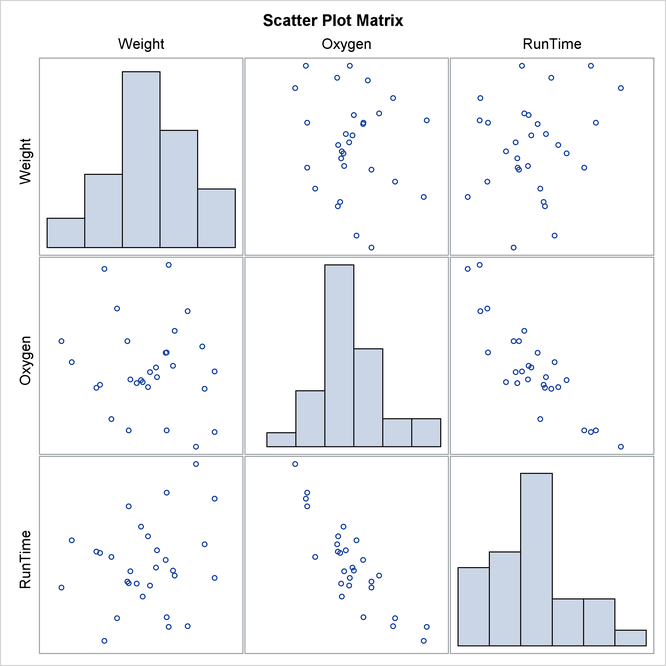### Example 2.1 Computing Four Measures of Association

This example produces a correlation analysis with descriptive statistics and four measures of association: the Pearson product-moment correlation, the Spearman rank-order correlation, Kendall’s tau-b coefficients, and Hoeffding’s measure of dependence,.

The `Fitness` data set created in the section Getting Started: CORR Procedure contains measurements from a study of physical fitness of 31 participants. The following statements request all four measures of association for the variables `Weight`, `Oxygen`, and `Runtime`:

```ods graphics on;
title 'Measures of Association for a Physical Fitness Study';
proc corr data=Fitness pearson spearman kendall hoeffding
plots=matrix(histogram);
var Weight Oxygen RunTime;
run;
ods graphics off;
```

Note that Pearson correlations are computed by default only if all three nonparametric correlations (SPEARMAN, KENDALL, and HOEFFDING) are not specified. Otherwise, you need to specify the PEARSON option explicitly to compute Pearson correlations.

The Simple Statistics table in Output 2.1.1 displays univariate descriptive statistics for analysis variables. By default, observations with nonmissing values for each variable are used to derive the univariate statistics for that variable. When nonparametric measures of association are specified, the procedure displays the median instead of the sum as an additional descriptive measure.

Output 2.1.1: Simple Statistics

 Measures of Association for a Physical Fitness Study

The CORR Procedure

3 Variables: Weight Oxygen RunTime

Simple Statistics
Variable N Mean Std Dev Median Minimum Maximum
Weight 31 77.44452 8.32857 77.45000 59.08000 91.63000
Oxygen 29 47.22721 5.47718 46.67200 37.38800 60.05500
RunTime 29 10.67414 1.39194 10.50000 8.17000 14.03000

The Pearson Correlation Coefficients table in Output 2.1.2 displays Pearson correlation statistics for pairs of analysis variables. The Pearson correlation is a parametric measure of association for two continuous random variables. When there are missing data, the number of observations used to calculate the correlation can vary.

Output 2.1.2: Pearson Correlation Coefficients

Pearson Correlation Coefficients
Prob > |r| under H0: Rho=0
Number of Observations
Weight Oxygen RunTime
Weight
 1.00000 31
 -0.15358 0.4264 29
 0.20072 0.2965 29
Oxygen
 -0.15358 0.4264 29
 1.00000 29
 -0.86843 <.0001 28
RunTime
 0.20072 0.2965 29
 -0.86843 <.0001 28
 1.00000 29

The table shows that the Pearson correlation between `Runtime` and `Oxygen` is0.86843, which is significant with a-value less than 0.0001. This indicates a strong negative linear relationship between these two variables. As `Runtime` increases, `Oxygen` decreases linearly.

The Spearman rank-order correlation is a nonparametric measure of association based on the ranks of the data values. The Spearman Correlation Coefficients table in Output 2.1.3 displays results similar to those of the Pearson Correlation Coefficients table in Output 2.1.2.

Output 2.1.3: Spearman Correlation Coefficients

Spearman Correlation Coefficients
Prob > |r| under H0: Rho=0
Number of Observations
Weight Oxygen RunTime
Weight
 1.00000 31
 -0.06824 0.725 29
 0.13749 0.4769 29
Oxygen
 -0.06824 0.725 29
 1.00000 29
 -0.80131 <.0001 28
RunTime
 0.13749 0.4769 29
 -0.80131 <.0001 28
 1.00000 29

Kendall’s tau-b is a nonparametric measure of association based on the number of concordances and discordances in paired observations. The Kendall Tau b Correlation Coefficients table in Output 2.1.4 displays results similar to those of the Pearson Correlation Coefficients table in Output 2.1.2.

Output 2.1.4: Kendall’s Tau-b Correlation Coefficients

Kendall Tau b Correlation Coefficients
Prob > |tau| under H0: Tau=0
Number of Observations
Weight Oxygen RunTime
Weight
 1.00000 31
 -0.00988 0.9402 29
 0.06675 0.6123 29
Oxygen
 -0.00988 0.9402 29
 1.00000 29
 -0.62434 <.0001 28
RunTime
 0.06675 0.6123 29
 -0.62434 <.0001 28
 1.00000 29

Hoeffding’s measure of dependence,, is a nonparametric measure of association that detects more general departures from independence. Without ties in the variables, the values of thestatistic can vary betweenand, withindicating complete dependence. Otherwise, thestatistic can result in a smaller value. The Hoeffding Dependence Coefficients table in Output 2.1.5 displays Hoeffding dependence coefficients. Since ties occur in the variable `Weight`, thestatistic for the `Weight` variable is less than.

Output 2.1.5: Hoeffding’s Dependence Coefficients

Hoeffding Dependence Coefficients
Prob > D under H0: D=0
Number of Observations
Weight Oxygen RunTime
Weight
 0.97690 <.0001 31
 -0.00497 0.5101 29
 -0.02355 1 29
Oxygen
 -0.00497 0.5101 29
 1.00000 29
 0.23449 <.0001 28
RunTime
 -0.02355 1 29
 0.23449 <.0001 28
 1.00000 29

When you use the PLOTS=MATRIX(HISTOGRAM) option, the CORR procedure displays a symmetric matrix plot for the analysis variables listed in the VAR statement (Output 2.1.6).

Output 2.1.6: Symmetric Scatter Plot MatrixThe strong negative linear relationship between `Oxygen` and `Runtime` is evident in Output 2.1.6.

Note that this graphical display is requested by enabling ODS Graphics and by specifying the PLOTS= option. For more information about ODS Graphics, see Chapter 21: Statistical Graphics Using ODS in SAS/STAT User's Guide.